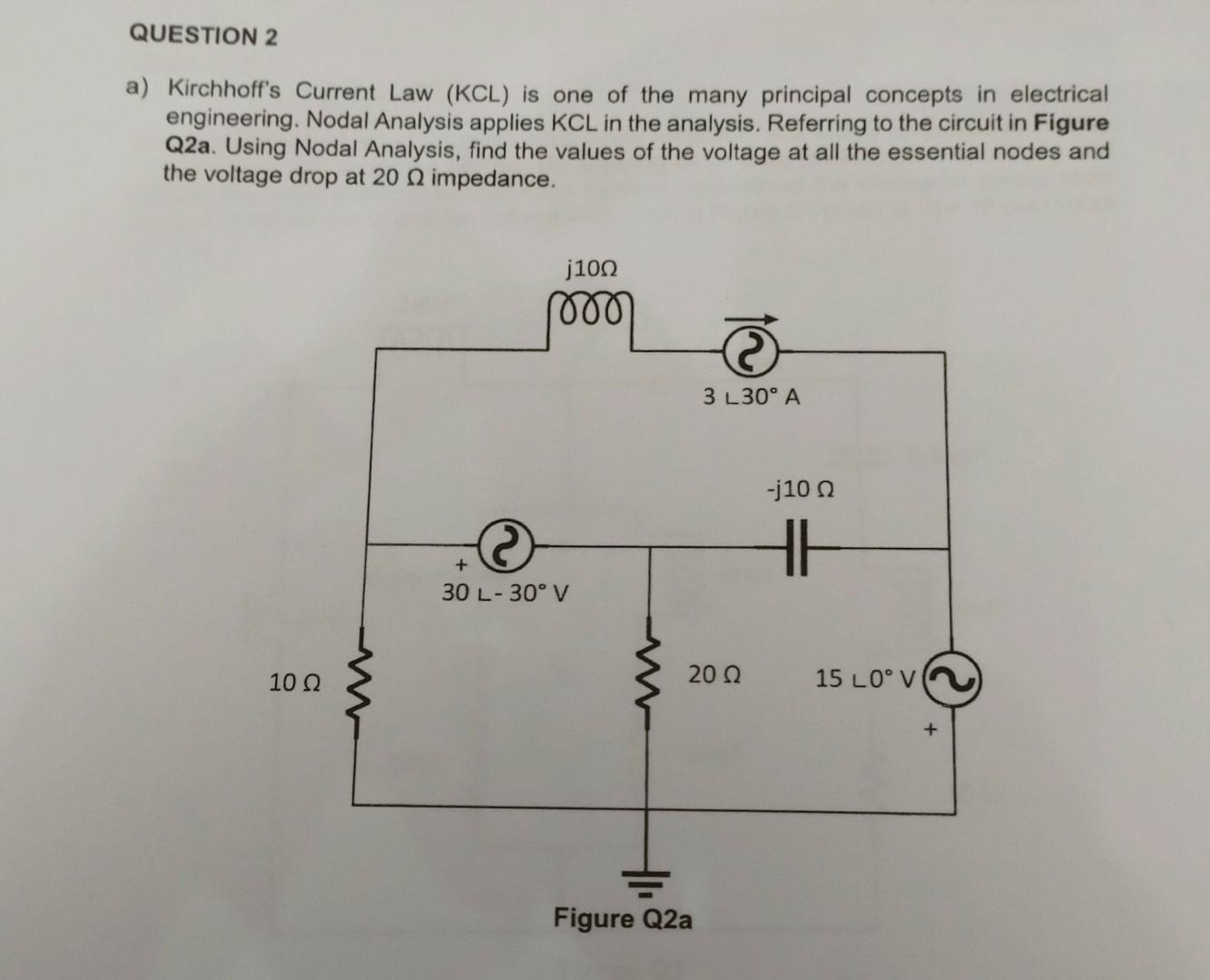Home / Expert Answers / Electrical Engineering / a-kirchhoff-39-s-current-law-kcl-is-one-of-the-many-principal-concepts-in-electrical-engineerin-pa406

# (Solved): a) Kirchhoff's Current Law (KCL) is one of the many principal concepts in electrical engineerin ...a) Kirchhoff's Current Law (KCL) is one of the many principal concepts in electrical engineering. Nodal Analysis applies KCL in the analysis. Referring to the circuit in Figure Q2a. Using Nodal Analysis, find the values of the voltage at all the essential nodes and the voltage drop at impedance.

We have an Answer from Expert